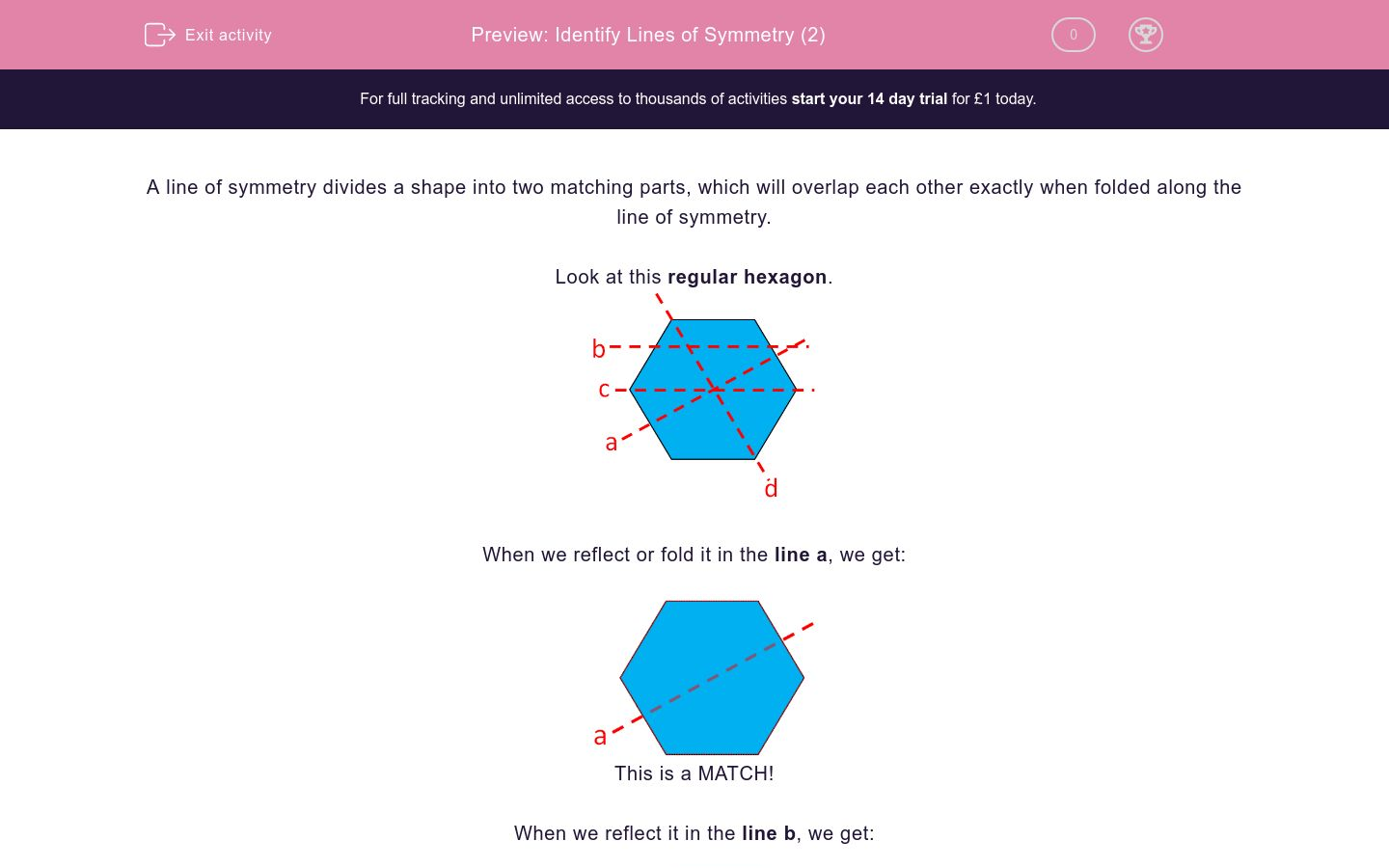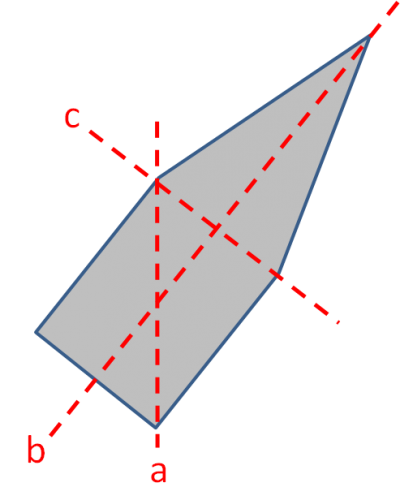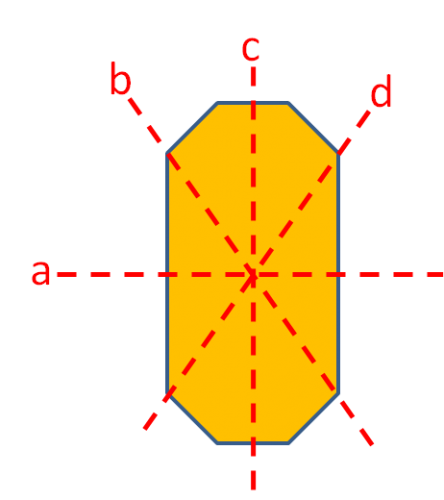# Identify Lines of Symmetry (2)

In this worksheet, students must identify the lines of symmetry for the given 2D shapes.Key stage:  KS 2

Curriculum topic:   Maths and Numerical Reasoning

Curriculum subtopic:   Symmetry

Difficulty level:### QUESTION 1 of 10

A line of symmetry divides a shape into two matching parts, which will overlap each other exactly when folded along the line of symmetry.

Look at this regular hexagon.When we reflect or fold it in the line a, we get:This is a MATCH!

When we reflect it in the line b, we get:This is not a MATCH!

When we reflect it in the line c, we get:This is a MATCH!

When we reflect it in the line d, we get:This is a MATCH!

So lines a, c and d are lines of symmetry.

Of course, a regular hexagon has a total of 6 lines of symmetry, but not all of them were shown.

Look at this isosceles trapezium.

Which of the lines are lines of symmetry?a

b

c

d

Look at this ellipse.

Which of the lines are lines of symmetry?a

b

c

d

Look at this rhombus.

Which of the lines are lines of symmetry?a

b

c

d

Look at this pentagon.

Which of the lines are lines of symmetry?a

b

c

none of these

Look at this regular hexagon.

Which of the lines are lines of symmetry?a

b

c

d

Look at this regular pentagon.

Which of the lines are lines of symmetry?a

b

c

d

Look at this octagon.

Which of the lines are lines of symmetry?a

b

c

d

Look at this star.

Which of the lines are lines of symmetry?a

b

c

d

e

Look at this cross.

Which of the lines are lines of symmetry?a

b

c

d

Look at this regular star (pentagram).

Which of the lines are lines of symmetry?a

b

c

d

e

• Question 1

Look at this isosceles trapezium.

Which of the lines are lines of symmetry?b
EDDIE SAYS
An isosceles trapezium only has one line of symmetry joining the midpoints of its parallel sides.
• Question 2

Look at this ellipse.

Which of the lines are lines of symmetry?a
b
EDDIE SAYS
The \'diagonals\' are not lines of symmetry.
• Question 3

Look at this rhombus.

Which of the lines are lines of symmetry?c
d
EDDIE SAYS
The diagonals of a rhombus cut each other at right angles and are the only lines of symmetry.
• Question 4

Look at this pentagon.

Which of the lines are lines of symmetry?b
EDDIE SAYS
Line b is the only fold that will give a match.
• Question 5

Look at this regular hexagon.

Which of the lines are lines of symmetry?b
d
EDDIE SAYS
The 6 lines of symmetry of a regular hexagon go from the middle of each side to a matching point on the opposite side, or from corner to corner.
• Question 6

Look at this regular pentagon.

Which of the lines are lines of symmetry?b
d
EDDIE SAYS
The 5 lines of symmetry of a regular pentagon go from each corner to the midpoint of the opposite side.
• Question 7

Look at this octagon.

Which of the lines are lines of symmetry?a
c
EDDIE SAYS
Lines a and c are the only ones which will give a match.
The \'diagonal\' lines will not work.
• Question 8

Look at this star.

Which of the lines are lines of symmetry?e
EDDIE SAYS
Line e is the only line of symmetry.
Look at the lengths of the lines if you are not sure.
• Question 9

Look at this cross.

Which of the lines are lines of symmetry?a
EDDIE SAYS
Line a is the only line of symmetry.
Look at the lengths of the lines if you are not sure.
• Question 10

Look at this regular star (pentagram).

Which of the lines are lines of symmetry?a
b
c
d
e
EDDIE SAYS
A regular pentagram has 5 lines of symmetry from each point to the opposite \'indent\'.
---- OR ----

Sign up for a £1 trial so you can track and measure your child's progress on this activity.

### What is EdPlace?

We're your National Curriculum aligned online education content provider helping each child succeed in English, maths and science from year 1 to GCSE. With an EdPlace account you’ll be able to track and measure progress, helping each child achieve their best. We build confidence and attainment by personalising each child’s learning at a level that suits them.

Get started